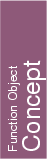## Kernel::ConstructCircumcenter_3

A model for this must provide:

Kernel::Point_3
 fo.operator() ( Kernel::Point_3 p, Kernel::Point_3 q, Kernel::Point_3 r, Kernel::Point_3 s)
compute the center of the sphere passing through the points p, q, r, and s.
Precondition: p, q, r, and s are not coplanar.

Kernel::Point_3 fo.operator() ( Kernel::Tetrahedron_3 t)
compute the center of the sphere passing through the vertices of t.
Precondition: t is not degenerate.

Kernel::Point_3
 fo.operator() ( Kernel::Point_3 p, Kernel::Point_3 q, Kernel::Point_3 r)
compute the center of the circle passing through the points p, q and r.
Precondition: p, q and r are not collinear.

Kernel::Point_3 fo.operator() ( Kernel::Triangle_3 t)
compute the center of the circle passing through the vertices of t.
Precondition: t is not degenerate.

### Refines

AdaptableFunctor (with four arguments)